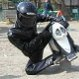Random Select objects from selected group lisp.

Recommended PostsHello,

I need a lisp that would randomly select a certain percentage of objects from an user pre-selected group. We are creating shutters, that are randomly perforated - don't want to manually randomly delete hundreds of small circles.

I've found a lisp from David Bethel (in this forum's archive: http://www.cadtutor.net/forum/archive/index.php/t-52841.html) which works fine, but it would select between all objects in the drawing, even on frozen layers.

The thread is very old so I don't know if Mr. Bethel is still active on this forum.

How would I change this lisp, so that it would first promp me to select objects from which it would then randomly select a certain percentage?

Thanks,

David

Share on other sitesHi there.

I think David is still active, hoping he wont mind.

Here'S what you need. Using the implied "_I" filter instead of "x".

(good example of wanting to highlight a selection set too...)

```(defun c:randset (/ ss sl i en el ep pct qty rs)

(defun randnum (/ modulus multiplier increment random)
(if (not seed)
(setq seed (getvar "DATE")))
(setq modulus    65536
multiplier    25173
increment    13849
seed       (rem (+ (* multiplier seed) increment) modulus)
random     (/ seed modulus)))

(cond ((setq ss (ssget "i"))
(sssetfirst nil nil)
(setq sl (sslength ss)
i -1)
(while (setq en (ssname ss (setq i (1+ i))))
(setq el (cons en el))))
(exit)))

(initget 7)
(setq pct (getreal "\nPercentage To Randomly Choose:   "))
(setq qty (fix (* sl pct 0.01)))

(while (> qty (sslength rs))
(setq ep (fix (* sl (randnum)))
en (nth ep el))
(if (not (ssmemb en rs))

(sssetfirst nil rs)
(princ)
)
```

That will form the selection you need.

Cheers

•1

Share on other sitesOn 5/2/2017 at 7:15 PM, Jef! said:

Hi there.

I think David is still active, hoping he wont mind.

Here'S what you need. Using the implied "_I" filter instead of "x".

(good example of wanting to highlight a selection set too...)﻿

﻿

```
(defun c:randset (/ ss sl i en el ep pct qty rs)

(defun randnum (/ modulus multiplier increment random)
(if (not seed)
(setq seed (getvar "DATE")))
(setq modulus    65536
multiplier    25173
increment    13849
seed       (rem (+ (* multiplier seed) increment) modulus)﻿
random     (/ seed modulus)))

(cond ((setq ss (ssget "i"))
(sssetfirst nil nil)
(setq sl (sslength ss)
i -1)
(while (setq en (ssname ss (setq i (1+ i))))
(setq el (cons en el))))
﻿       (T (alert "\nNo Entities Found")
(exit)))

(initget 7)﻿
(setq pct (getreal "\nPercentage To Randomly Choose:   "))
(setq qty (fix (* sl pct 0.01)))

(while (> qty (sslength rs))
(setq ep (fix (* sl (randnum)))﻿
en (nth ep el))
(if (not (ssmemb en rs))

(sssetfirst nil rs)
(princ)
)﻿
```

That will form the selection you need.

Cheers

Can this be modified to ask for number of objects to be selected, instead of percentage?

Share on other sitesPosted (edited)

>> Can this be modified to ask for number of objects to be selected, instead of percentage?

``` (setq pct﻿ ﻿(getreal ﻿"\nPercentage To Randomly Choose:   "))
(setq qty (fix (* sl pct 0.01)))﻿```

do this:

```
(setq qty (geting "\nNumber of items to be selected: "))

```

Edited by Emmanuel Delay
•1

Join the conversation

You can post now and register later. If you have an account, sign in now to post with your account.
Note: Your post will require moderator approval before it will be visible.×   Pasted as rich text.   Paste as plain text instead

Only 75 emoji are allowed.